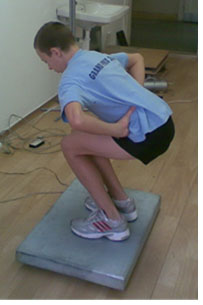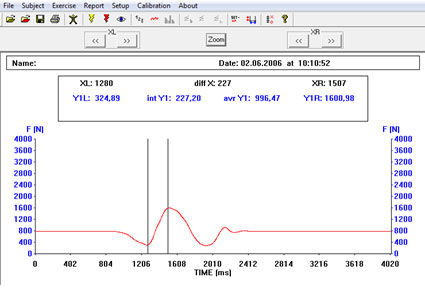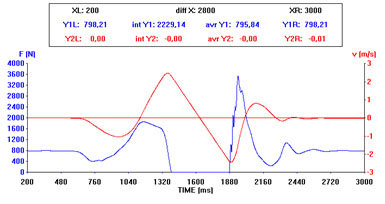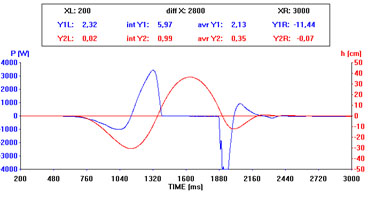A computer based diagnostic system for the assessment of strength capabilities consisting of strain gauge force plate (one-, or two-dimensional), electronics, 12-bit AD convertor, interface and software.Software consists of data aquisition and storage, calibration, and extensive analytic reporting (time zooming with integral a mean calculation from defined time segments for parameter assigned to Y1 and Y2 axes).Software also includes a special analytical module for calculation of acceleration, velocity, displacement and power from force-time curve (see below) during single squat or jump.

Calculation of additional biomechanical parameters from force-time curve: basic principle.

Verical force (F) applied to the plate consists of weight (product of body mass m and gravitational constant g) and inertia component (product of body mass m and vertical acceleration a)

F =  (m  x  g) + (m  x  a)

F =  m  x  (g + a)

F

a  =  –––  –  g

m

v =  integral of a   (a . t)

h =  integral of v   (v . t)

P =  F x  vMaximal power: 3455 W and 43,2 W/kg,  resp.

Height of the jump:  36.7 cm

## Testing Protocol

–       Squat jump

–       Counter movement jump

–       Drop jump

–       Jumps (squat, counter mobement and drop) with additional weight

## Parameters

– Mean and maximal power during take off (W)

– Height of the jump (m/s)

## Applications

–       assessment of strength capabilities of lower extremities

–       evaluation the effect of training